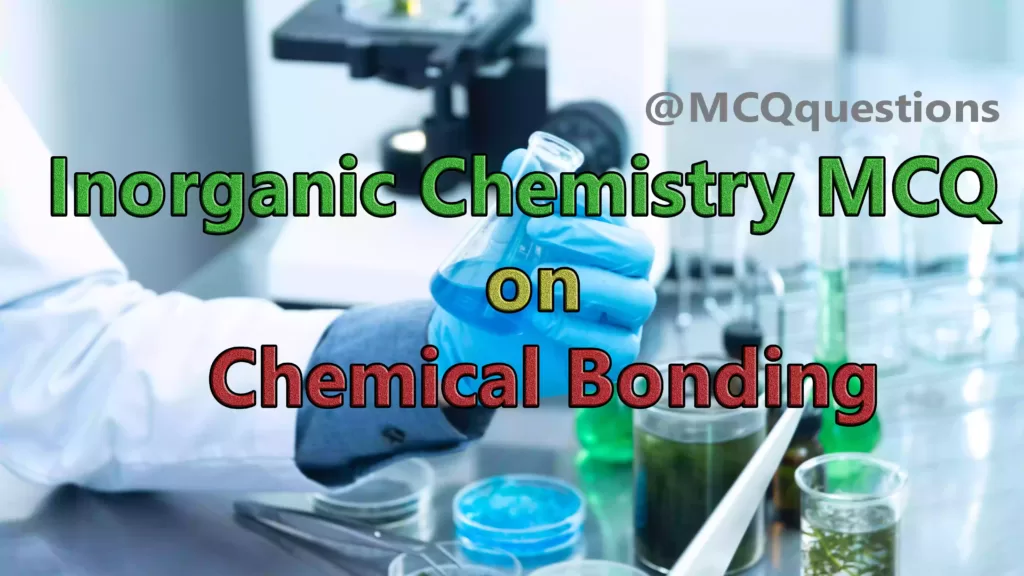# Inorganic Chemistry MCQ on Chemical Bonding

## Inorganic Chemistry MCQ on Chemical Bonding

1. Multiple covalent bond containing compound is

(a) CH4

(b) S8

(c) P4

(d) H2

`Answer (c) P4`

2. The octet rule is observed in

(a) PCI5

(b) CO2

(c) BCI3

(d) SF6

`Answer (b) CO2`

3. The van der Waal’s force occurs in

(a) Liquid HF

(b) Liquid NH,

(c) Ice

(d) Liquid argon

`Answer (d) Liquid argon`

4. The compound containing coordinate bond is

(a) SO3

(b) H2SO4

(c) O3

(d) all of these

`Answer (d) all of these`

5. Which among the following does not have the hydrogen bond?

(a) Water

(b) Liquid NH

(c) Phenol

(d) Liquid HCL

`Answer (d) Liquid HCL`

6. Carbon tetrachloride has no dipole moment, because

(a) Similar sizes of carbon and chlorine atoms

(b) It is symmetrical tetrahedral structure

(c) It is planer structure

(d) None of these.

`Answer (b) It is symmetrical tetrahedral structure`

7. The tetrahedral structure is possessed by

(a) BF4-

(b) CH4

(c) NH4+

(d) all of these

`Answer (d) all of these`

8. In diamond, the bond present is

(a) Hydrogen

(b) Covalent

(c) Electrovalent

(d) Coordinate bond.

`Answer (b) Covalent`

9. The bond angle of sp²-hybrid orbital is

(a) 105°

(b) 120°

(c) 180°

(d) 109°28′

`Answer (b) 120°`

10. The types of bond present in CuSO4. 5H2O are only

(a) Electrovalent and covalent

(b) Electrovalent and coordinate covalent

(c) Electrovalent, covalent and coordinate-covalent

(d) Electrovalent, covalent and hydrogen.

`Answer (a) Electrovalent and covalent`

11. Molecular shape of PF5, is

(a) square planar

(b) Trigonal bipyramidal

(c) Tetrahedral three-dimensional

(d) Octahedral three-dimensional.

`Answer (b) Trigonal bipyramidal`

12. Ionic bond can easily be formed between

(a) H and O

(b) H and Cl

(c) H and N

(d) Na and Cl

`Answer (d) Na and Cl`

13. What happend in presence of more electronegative atom to the strength of ionic bond?

(a) Decreases

(b) Increases

(c) remains same

(d) Any one of these.

`Answer (b) Increases`

14. If we consider the ammonia molecule to be sp³ hybridised. the lone pair will occupy

(a) An s-orbital

(b) A p-orbital

(c) A sp²-hybridised orbital

(d) A sp³-hybridised orbital

`Answer (d) A sp³-hybridised orbital`

15. Strongest hydrogen bonds in vapour phase are found in

(a) HF—– HF

(b) HCI—–HCI

(c) HF—- HCI

(d) HF—-HI.

`Answer (a) HF----- HF`

16. Good electron pair donor

Is

(a) P(CH3)3

(b) PH3

(c) N(CH3,)3

(d) NH3

`Answer (c) N(CH3,)3`

17. Write the state of hybridization in PCL5

(a) dsp³

(b) sp³d

(c) dsp²

(d) sp³d²

`Answer (b) sp³d`

18. Which of the following has smallest bond angle?

(a) H2O

(b) NH3

(c) CH4

(d)CO2

`Answer (a) H2O`

19. Sulphate ion is

(a) square planar

(b) Pyramidal

(c) Rhombic

(d) Tetrahedral

`Answer (d) Tetrahedral`

20. The correct order of lattice energies is

(a) NaCl > MgBr2 > CaO > Al2O3

(b) NaCl > CaO > MgBr2 > Al2O3

(c) Al2O3 > MgBr2 > CaO > NaCl

(d) Al2O3 > CaO > MgBr2 > NaCl

`Answer (d) Al2O3 > CaO > MgBr2 > NaCl`

21. The bond angle in water is

(a) 120°

(b) 107°

(c) 109.5°

(d) 104.5°

`Answer (d) 104.5°`

22. Which of the following molecule is planar?

(a) CH4

(b) C2H4

(c) NH3

(d) SiC14

`Answer (b) C2H4`

23. Sulphuric acid provides a simple example of

(a) Coordinate bonds

(b) Non-covalent compound

(c) Covalent ion.

(d) None of these.

`Answer (d) None of these.`

24. The interionic attraction depends on interaction of

(a) Solute solute

(b) The charges

(c) Solvent solvent

(d) Molecular properties

`Answer (b) The charges`

25. The pair of having similar geometry is

(a) BF,3,NH3

(b) H2O, C2H2

(c) CO2, SO2

(d) NH3 and PH3

`Answer (d) NH3 and PH3`

26. The strongest hydrogen bonding  in its liquid phase is observed in

(a) H-F

(b) HI

(c) CH4

(d) PH3

`Answer (a) H-F`

27. Maximum polarity found in

(a) C-O

(b) C – Br

(c) C-S

(d) C-F

`Answer (d) C-F`

28. According to VSEPR theory, BeF, molecule is

(a) Planar

(b) Linear

(c) Tetrahedral

(d) Pyramidal.

`Answer (b) Linear`

29. The stability of an ionic compound ie because of

(a) Lattice energy

(b) Electron affinity

(c) Ionization energy

(d) Electronegativity.

`Answer (a) Lattice energy`

30. The small hybridised carbon atom bond  is

(a) Propane

(b) Propene

(c) Butane

(d) Propyne

`Answer (d) Propyne`

31. The hybridisation of carbon in CO2 is

(a) sp

(b) Sp²

(c) Sp³

(d) None

`Answer (a) sp`

32. Which of the following has sp²-hybridisation?

(a) C2H6

(b) C2H4

(c) BeCl2

(d) C2H2

`Answer (b) C₂H4`

Also You Can Read Inorganic Chemistry MCQ